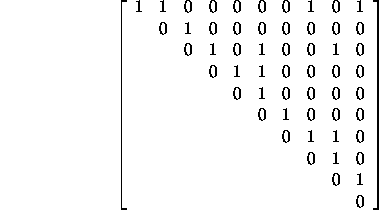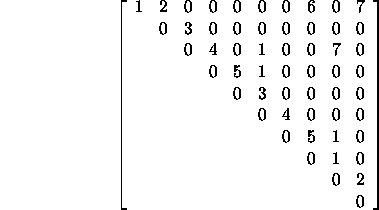CSE 5311 Section 501 Fall 1998
Homework 5
Due: April 6, 1998, in class

1. List the vertices in each connected component after each iteration of the second loop in the Connected-Components code when applied to graph G. Graph G = (V, E), where V = {a, b, c, d, e, f, g, h, i, j} and edges in E = {(a,c), (a,b), (e,f), (h,i), (e,g), (a,d), (e,d), (b,d), (c,a), (h,j)} are processed in the order listed.

```Edge processed |                 Disjoint sets
---------------|----------------------------------------------------------------
initial     | {a} {b} {c} {d} {e} {f} {g} {h} {i} {j}
(a,c)       | {a,c} {b} {d} {e} {f} {g} {h} {i} {j}
(a,b)       | {a,b,c} {d} {e} {f} {g} {h} {i} {j}
(e,f)       | {a,b,c} {d} {e,f} {g} {h} {i} {j}
(h,i)       | {a,b,c} {d} {e,f} {g} {h,i} {j}
(e,g)       | {a,b,c} {d} {e,f,g} {h,i} {j}
(a,d)       | {a,b,c,d} {e,f,g} {h,i} {j}
(e,d)       | {a,b,c,d,e,f,g} {h,i} {j}
(b,d)       | {a,b,c,d,e,f,g} {h,i} {j}
(c,a)       | {a,b,c,d,e,f,g} {h,i} {j}
(h,j)       | {a,b,c,d,e,f,g} {h,i,j}```
2. Argue on the basis of Theorem 22.1 in the textbook that we can obtain amortized time bounds of O(1) for Make-Set and Find-Set and O(lg n) for Union using the linked-list representation and the weighted-union heuristic.

We want to show that we can assign O(1) charges to Make-Set and Find-Set and a O(lg n) charge to Union such that the charges for a sequence of these operations are enough to cover the cost of the sequence - O(m + nlgn), according to Theorem 22.1.

Consider the usual sequence of m Make-Set, Union, and Find-Set operations, n of which are Make-Set operations, and let l<n be the number of Union operations (l<n because there are only n sets available to combine). Then there are n Make-Set operations, l Union operations, and m-n-l Find-Set operations.

The theorem does not separately name the number l of Unions; rather, it bounds the number by n. If you go through the proof of the theorem with l Unions, you get the time bound O(m - l + l lg l) = O(m + l lg l) for the sequence of operations. Thus the sequence of operations takes <= c(m + l lg l) time for some constant c.

We want to assign operation charges such that
(Make-Set charge) * n
+ (Find-Set charge) * (m-n-l)
+ (Union charge) * l
= c(m + l lg l).

The following assignments work, where c' is some constant >= c:

• Make-Set -- c'
• Find-Set -- c'
• Union -- c'(lg n + 1)

Substituting into the above sum we get

c'n + c'(m-n-l) + c'(lgn + 1)l = c'm + c'llgn
= c'(m+llgn)
> c(m|llgl)
3. Consider the following adjacency matrix for an undirected graph:The 1s here indicate the presence of an edge. I want you to construct your own set of weights for each edge. To make sure you all have unique weights, use the non-zero digits from your Social Security Number, your birthday, and your phone number as weights, in that order (with repetition if necessary).

Show the minimum spanning tree that results from using Kruskal's algorithm and from using Prim's algorithm. Show each step of the procedure for both algorithms.

Consider as an example the graph using the following randomly-generated weights.MST-Kruskal sets:

```{a} {b} {c} {d} {e} {f} {g} {h} {i} {j}
{a} {b} {c} {d} {e} {f} {g} {h,i} {j}   A = {(h,i)}
{a} {b} {c,f} {d} {e} {g} {h,i} {j}   A = {(h,i), (c,f)}
{a,b} {c,f} {d} {e} {g} {h,i} {j}   A = {(h,i), (c,f), (a,b)}
{a,b} {c,d,f} {e} {g} {h,i} {j}   A = {(h,i), (c,f), (a,b), (d,f)}
{a,b} {c,d,f} {e} {g} {h,i,j}   A = {(h,i), (c,f), (a,b), (d,f), (i,j)}
{a,b,c,d,f} {e} {g} {h,i,j}   A = {(h,i), (c,f), (a,b), (d,f), (i,j), (b,c)}
{a,b,c,d,e,f} {g} {h,i,j}   A = {(h,i), (c,f), (a,b), (d,f), (i,j), (b,c),
(e,f)}
{a,b,c,d,e,f,g} {h,i,j}   A = {(h,i), (c,f), (a,b), (d,f), (i,j), (b,c),
(e,f), (f,g)}
{a,b,c,d,e,f,g,h,i,j}   A = {(h,i), (c,f), (a,b), (d,f), (i,j), (b,c),
(e,f), (f,g), (g,h)}```

MST-Prim

 Edge Priority Queue Q = (a 0, b, c, d, e, f, g, h, i, j) (a,b) Q = (b 2, c, d, e, f, g, h 6, i, j 7) (b,c) Q = (c 3, d, e, f, g, h 6, i, j 7) (c,f) Q = (d 4, e, f 1, g, h 6, i 7, j 7) (d,f) Q = (d 2, e 3, g 4, h 6, i 7, j 7) (e,f) Q = (e 3, g 4, h 6, i 7, j 7) (f,g) Q = (g 4, h 6, i 7, j 7) (g,h) Q = (h 5, i 7, j 7) (h,i) Q = (i 1, j 7) (i,j) Q = (j 2)

4. Show the order in which the nodes in graph G are visited (dequeued) using a Depth-First Search and a Breadth-First Search. G = {a, b, c, d, e, f, g} and E = {(a,b), (a,c), (b,d), (c,e), (d,f), (e,f), (e,g)}. Start at node a and check edges in alphabetical order.

BFS: a, b, c, d, e, f, g

DFS: a, b, d, f, e, c, g

5. Give a topological sort for the following relations: (a < b, a < c, d < b, e < c, a < e, a < d).

Element a must be first followed by d or e, b can be anywhere after d, and c could be anywhere after e. One possible order is a d e b c.